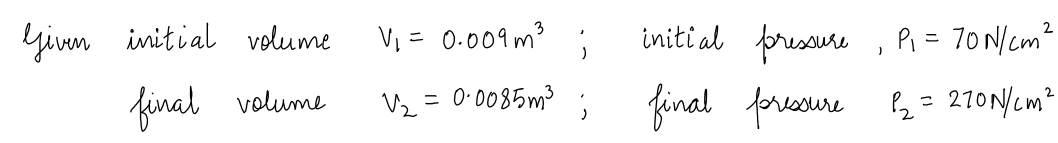# Determine the bulk modulus of elasticity of a fluid which is compressed in a cylinder from a volume of 0.009 m3 at 70 N/cm2 pressure to a volume of 0.0085 m² at 270 N/cm² pressure.

Question-AnswerCategory: Fluid MechanicsDetermine the bulk modulus of elasticity of a fluid which is compressed in a cylinder from a volume of 0.009 m3 at 70 N/cm2 pressure to a volume of 0.0085 m² at 270 N/cm² pressure.

Determine the bulk modulus of elasticity of a fluid which is compressed in a cylinder from a volume of 0.009 m3 at 70 N/cm2 pressure to a volume of 0.0085 m² at 270 N/cm² pressure.

Step 1Step 2Step 3